# Entanglement Spectrum Topological Entanglement Entropy and a Quantum

• Slides: 60Entanglement Spectrum, Topological Entanglement Entropy and a Quantum Hammersley-Clifford Theorem Fernando G. S. L. Brandão Microsoft Research based on joint work with Kohtaro Kato University of Tokyo MIT 2016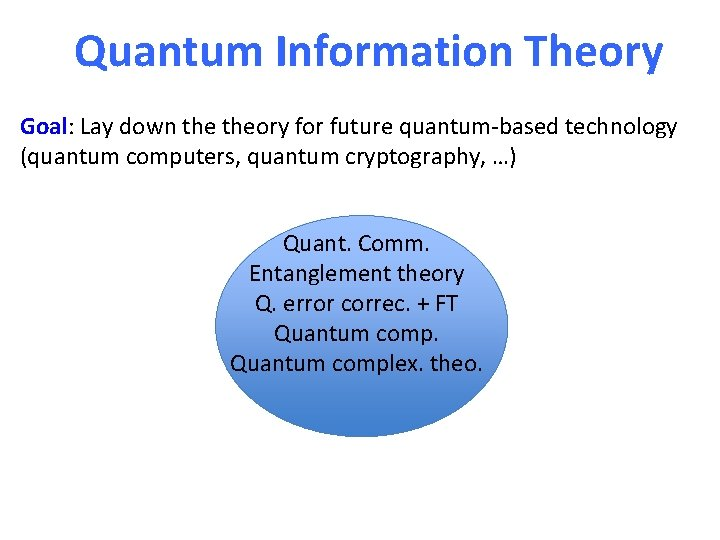Quantum Information Theory Goal: Lay down theory for future quantum-based technology (quantum computers, quantum cryptography, …) Quant. Comm. Entanglement theory Q. error correc. + FT Quantum complex. theo.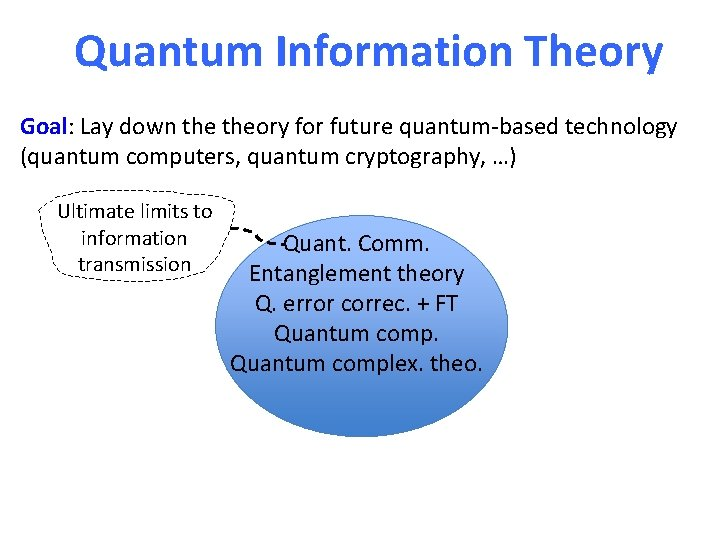Quantum Information Theory Goal: Lay down theory for future quantum-based technology (quantum computers, quantum cryptography, …) Ultimate limits to information transmission Quant. Comm. Entanglement theory Q. error correc. + FT Quantum complex. theo.Quantum Information Theory Goal: Lay down theory for future quantum-based technology (quantum computers, quantum cryptography, …) Ultimate limits to information transmission Entanglement as a resource Quant. Comm. Entanglement theory Q. error correc. + FT Quantum complex. theo.Quantum Information Theory Goal: Lay down theory for future quantum-based technology (quantum computers, quantum cryptography, …) Ultimate limits to information transmission Entanglement as a resource Quantum computers are digital Quant. Comm. Entanglement theory Q. error correc. + FT Quantum complex. theo.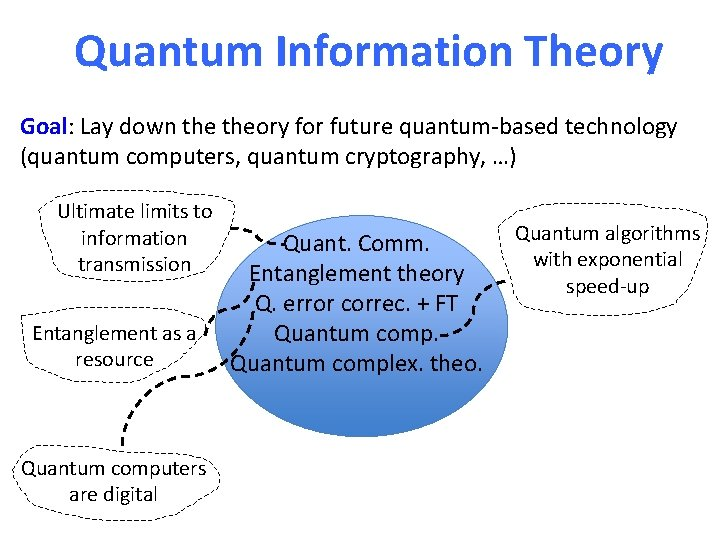Quantum Information Theory Goal: Lay down theory for future quantum-based technology (quantum computers, quantum cryptography, …) Ultimate limits to information transmission Entanglement as a resource Quantum computers are digital Quant. Comm. Entanglement theory Q. error correc. + FT Quantum complex. theo. Quantum algorithms with exponential speed-up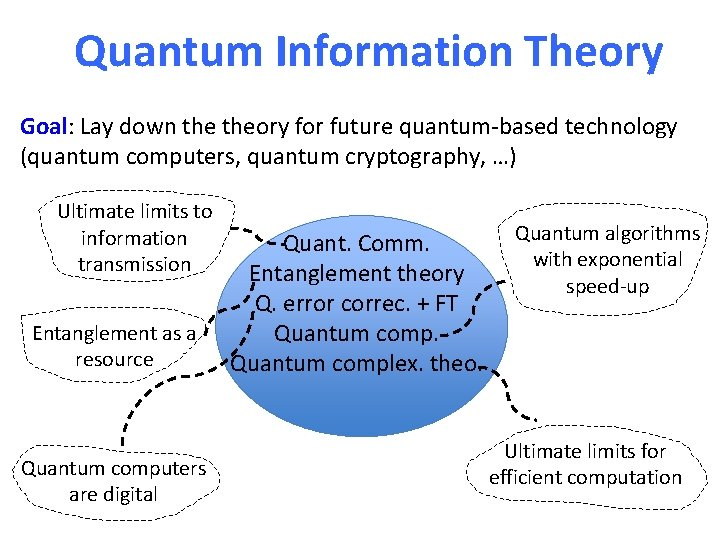Quantum Information Theory Goal: Lay down theory for future quantum-based technology (quantum computers, quantum cryptography, …) Ultimate limits to information transmission Entanglement as a resource Quantum computers are digital Quant. Comm. Entanglement theory Q. error correc. + FT Quantum complex. theo. Quantum algorithms with exponential speed-up Ultimate limits for efficient computation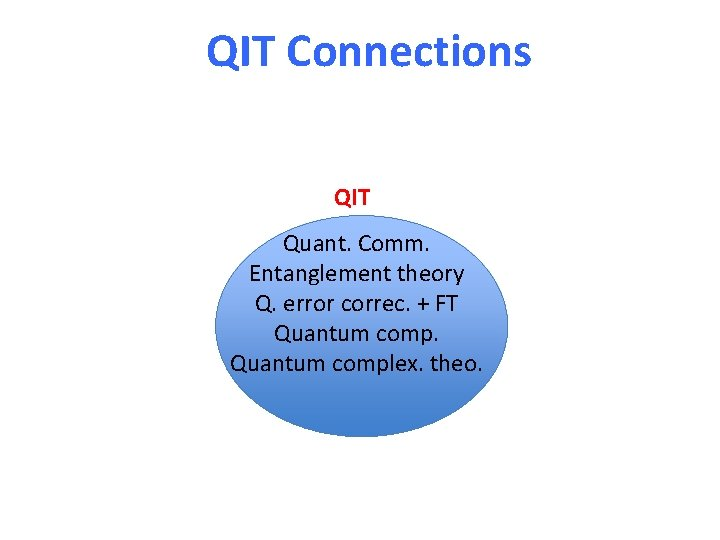QIT Connections QIT Quant. Comm. Entanglement theory Q. error correc. + FT Quantum complex. theo.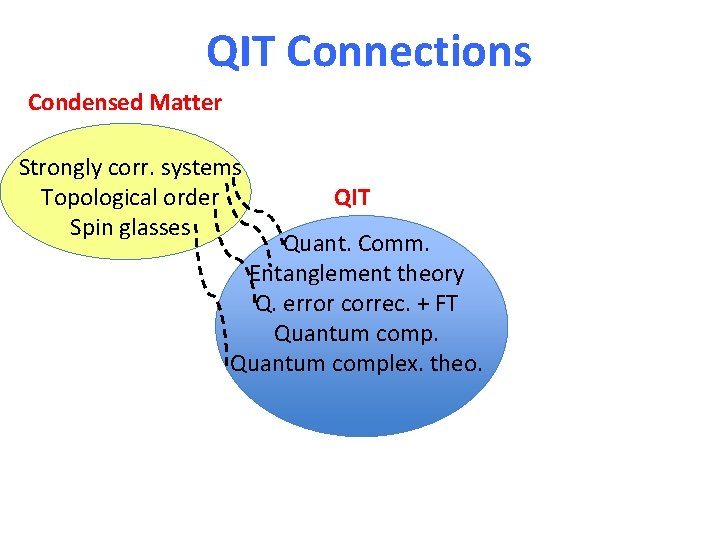QIT Connections Condensed Matter Strongly corr. systems Topological order Spin glasses QIT Quant. Comm. Entanglement theory Q. error correc. + FT Quantum complex. theo.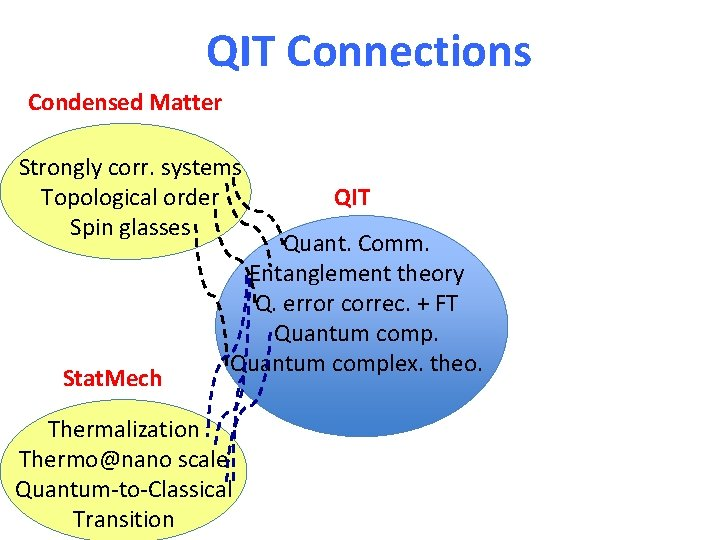QIT Connections Condensed Matter Strongly corr. systems Topological order Spin glasses Stat. Mech QIT Quant. Comm. Entanglement theory Q. error correc. + FT Quantum complex. theo. Thermalization [email protected] scale Quantum-to-Classical TransitionQIT Connections Condensed Matter HEP/GR Strongly corr. systems Topological order Spin glasses Topolog. q. field theo. Black hole physics Holography Stat. Mech QIT Quant. Comm. Entanglement theory Q. error correc. + FT Quantum complex. theo. Thermalization [email protected] scale Quantum-to-Classical TransitionQIT Connections Condensed Matter HEP/GR Strongly corr. systems Topological order Spin glasses Topolog. q. field theo. Black hole physics Holography Stat. Mech QIT Quant. Comm. Entanglement theory Q. error correc. + FT Quantum complex. theo. Thermalization [email protected] scale Quantum-to-Classical Transition Exper. Phys. Ion traps, linear optics, optical lattices, c. QED, superconduc. devices, many more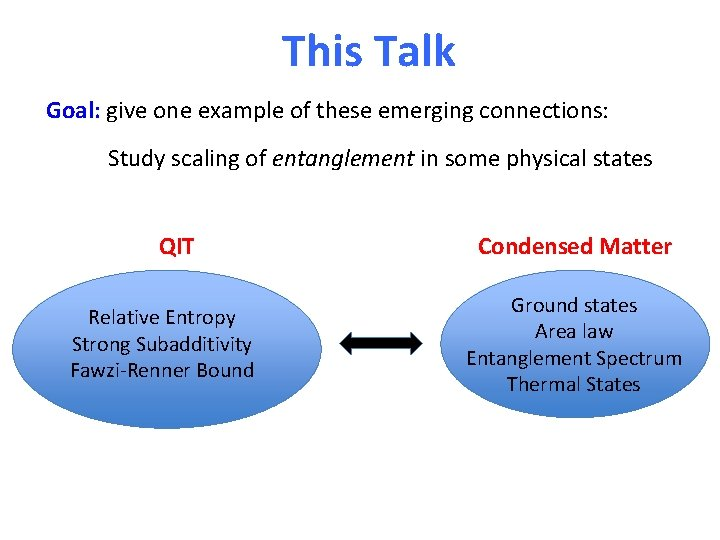This Talk Goal: give one example of these emerging connections: Study scaling of entanglement in some physical states QIT Condensed Matter Relative Entropy Strong Subadditivity Fawzi-Renner Bound Ground states Area law Entanglement Spectrum Thermal States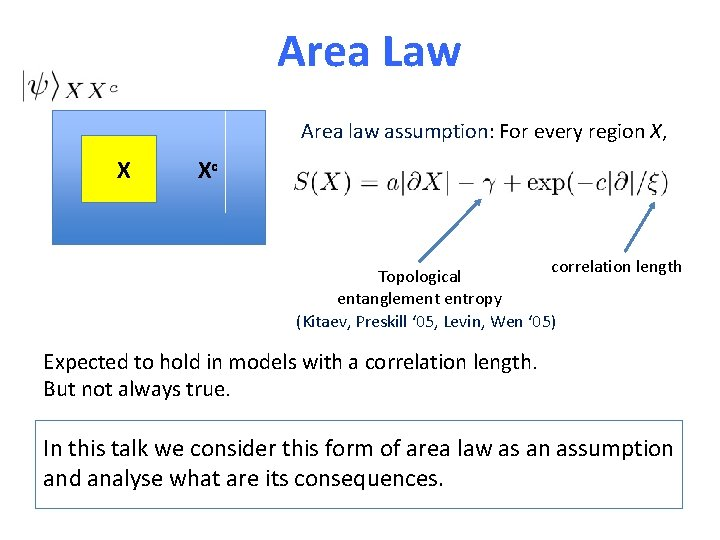Area Law Area law assumption: For every region X, X Xc correlation length Topological entanglement entropy (Kitaev, Preskill ‘ 05, Levin, Wen ‘ 05) Expected to hold in models with a correlation length. But not always true. In this talk we consider this form of area law as an assumption and analyse what are its consequences.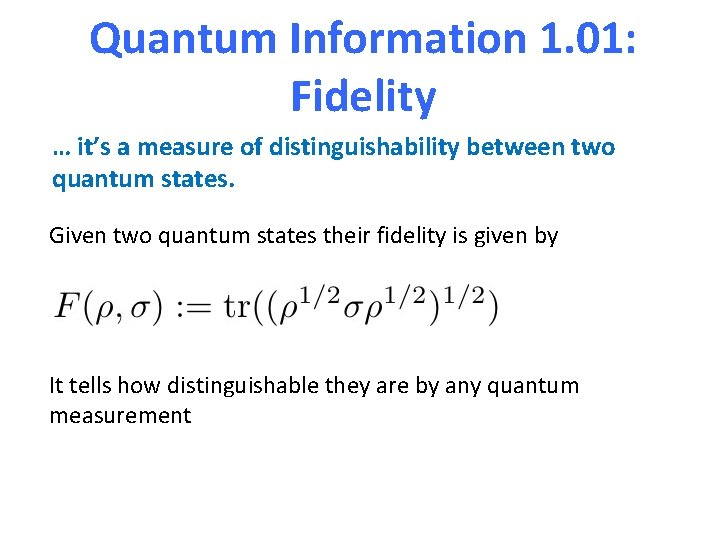Quantum Information 1. 01: Fidelity … it’s a measure of distinguishability between two quantum states. Given two quantum states their fidelity is given by It tells how distinguishable they are by any quantum measurementQuantum Information 1. 01: Relative Entropy … it’s another measure of distinguishability between two quantum states. Def: Gives optimal exponent for distinguishing the two states (in asymmetric hypothesis testing; Stein’s Lemma) Pinsker’s inequality: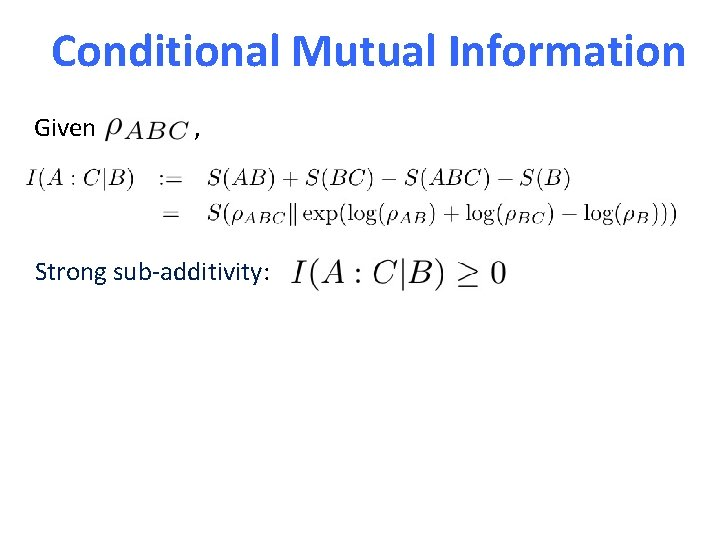Conditional Mutual Information Given , Strong sub-additivity:Conditional Mutual Information Given , Strong sub-additivity: Fawzi-Renner ‘ 14: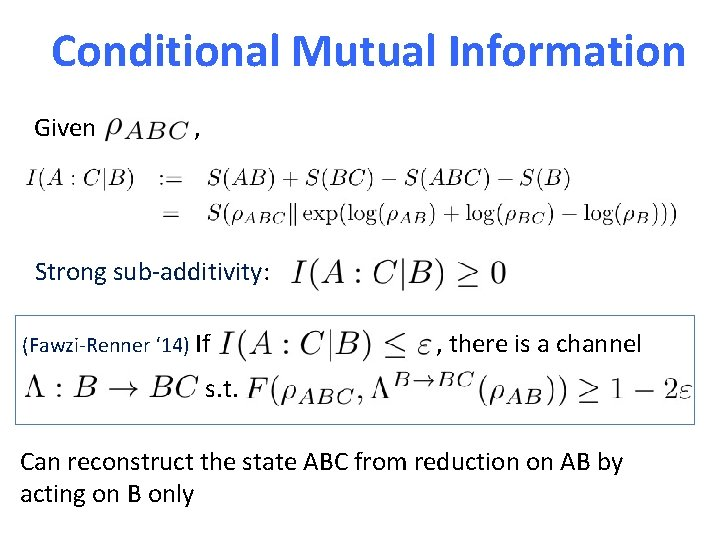Conditional Mutual Information Given , Strong sub-additivity: (Fawzi-Renner ‘ 14) If , there is a channel s. t. Can reconstruct the state ABC from reduction on AB by acting on B onlyConsequence of Area Law: State Reconstruction Area law assumption: For every region X, A B C l Topological entanglement entropy A B C correlation length For every ABC with trivial topology: (Kitaev ‘ 12) implies the state can be created by short-depth circuit (Kim ‘ 14) Implies the state can be constructed from local partsTopological Entanglement Entropy (Kitaev, Preskill ‘ 05, Levin, Wen ‘ 05) Area law assumption: For every region X, A B B l C Conditional Mutual Information: Assuming area law holds: Topological entanglement entropy correlation length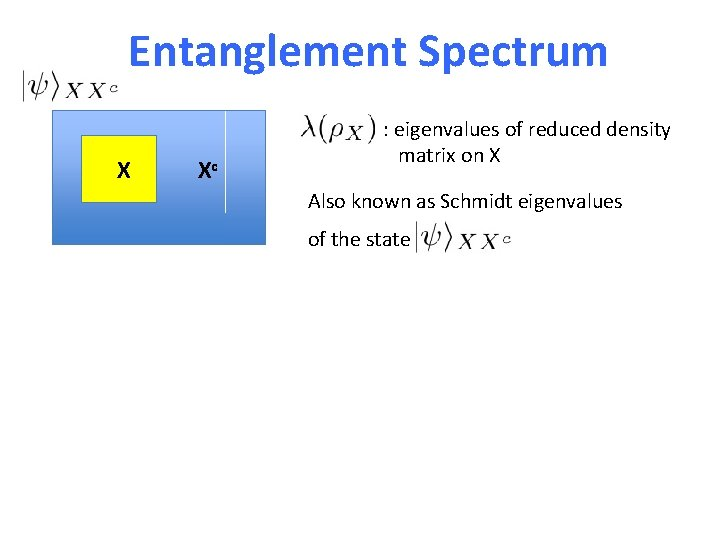Entanglement Spectrum X Xc : eigenvalues of reduced density matrix on X Also known as Schmidt eigenvalues of the state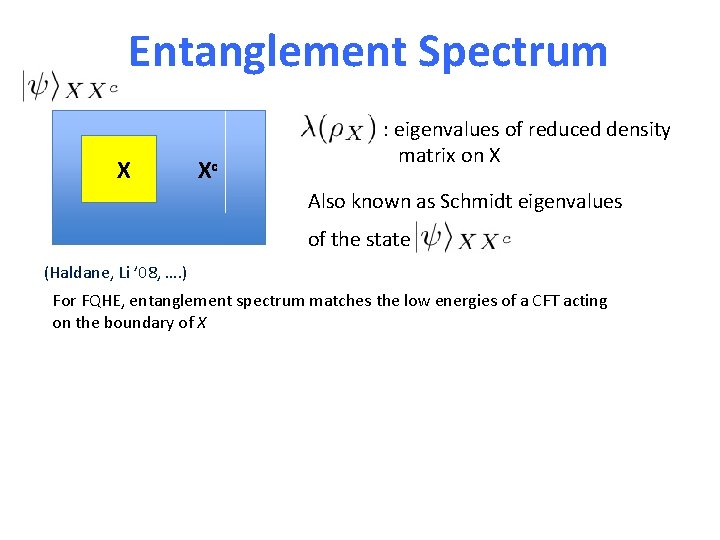Entanglement Spectrum X Xc : eigenvalues of reduced density matrix on X Also known as Schmidt eigenvalues of the state (Haldane, Li ’ 08, …. ) For FQHE, entanglement spectrum matches the low energies of a CFT acting on the boundary of XEntanglement Spectrum X : eigenvalues of reduced density matrix on X Xc Also known as Schmidt eigenvalues of the state (Haldane, Li ’ 08, …. ) For FQHE, entanglement spectrum matches the low energies of a CFT acting on the boundary of X (Cirac, Poiblanc, Schuch, Verstraete ’ 11, …. ) Numerical studies with PEPS. For topologically trivial systems (AKLT, Heisenberg models): entanglement spectrum matches the energies of a local Hamiltonian on boundary For topological systems (Toric code): needs non-local Hamiltonian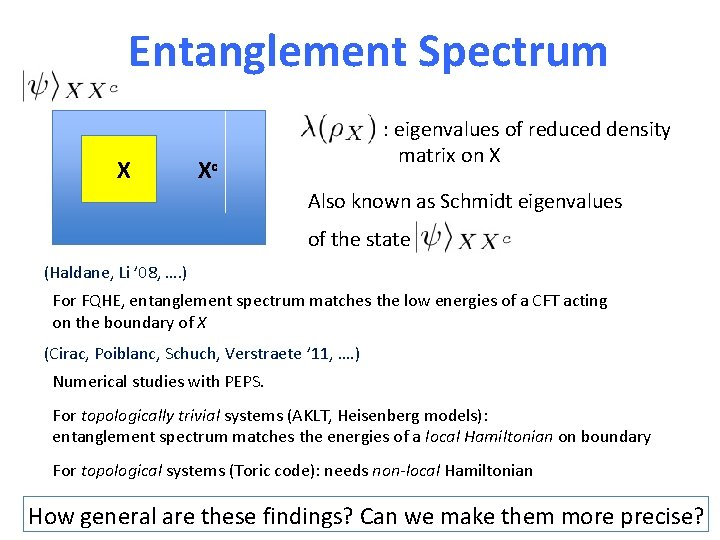Entanglement Spectrum X : eigenvalues of reduced density matrix on X Xc Also known as Schmidt eigenvalues of the state (Haldane, Li ’ 08, …. ) For FQHE, entanglement spectrum matches the low energies of a CFT acting on the boundary of X (Cirac, Poiblanc, Schuch, Verstraete ’ 11, …. ) Numerical studies with PEPS. For topologically trivial systems (AKLT, Heisenberg models): entanglement spectrum matches the energies of a local Hamiltonian on boundary For topological systems (Toric code): needs non-local Hamiltonian How general are these findings? Can we make them more precise?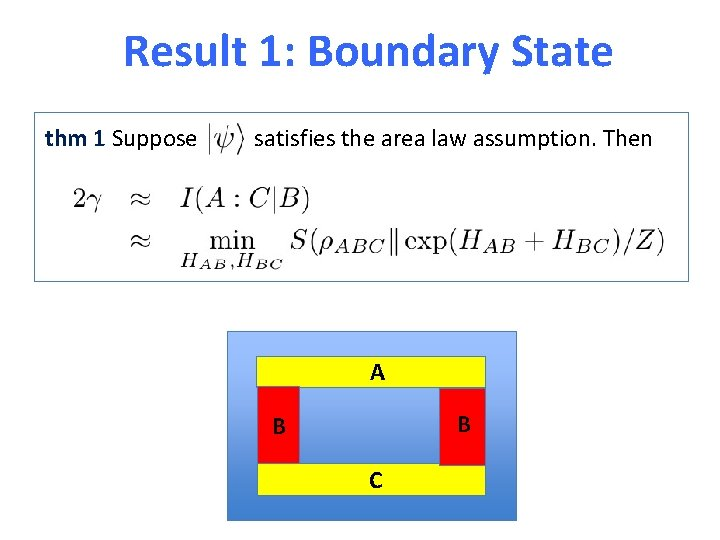Result 1: Boundary State thm 1 Suppose satisfies the area law assumption. Then A B B CResult 1: Boundary State thm 1 Suppose satisfies the area law assumption. Then Suppose . Then there is a local s. t. B 2 B 3 … Bk-2 Bk-1 B 2 k … Bk+2 Bk Bk+1Result 1: Boundary State thm 1 Suppose satisfies the area law assumption. Then Suppose . Then there is a local s. t. Local ”boundary Hamiltonian” Non-local ”boundary Hamiltonian”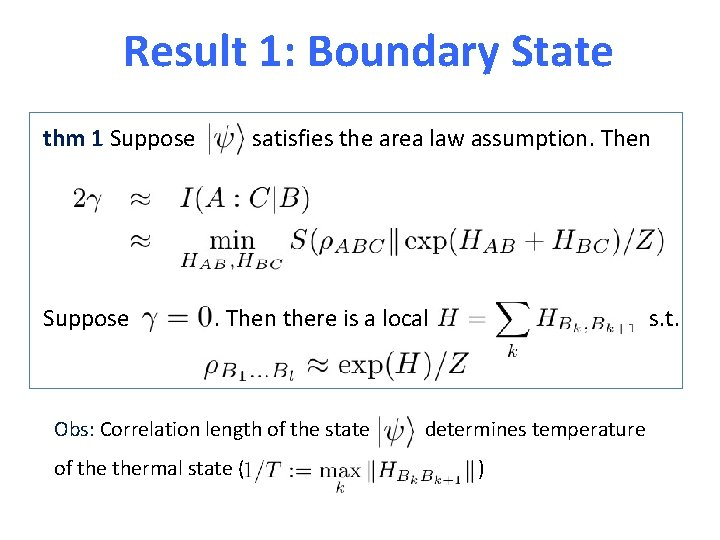Result 1: Boundary State thm 1 Suppose satisfies the area law assumption. Then Suppose . Then there is a local s. t. Obs: Correlation length of the state determines temperature of thermal state ( )Result 2: Entanglement Spectrum thm 2 Suppose satisfies the area law assumption with . Then … X B 1 B 2 B 3 Bl-1 Bl X’Result 2: Entanglement Spectrum thm 2 Suppose satisfies the area law assumption with . Then If , then for every k there is no local Hamilatonian H s. t.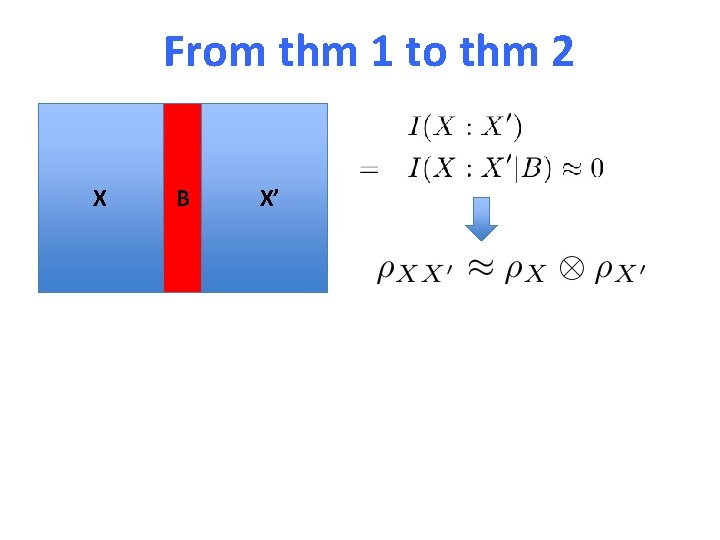From thm 1 to thm 2 X B X’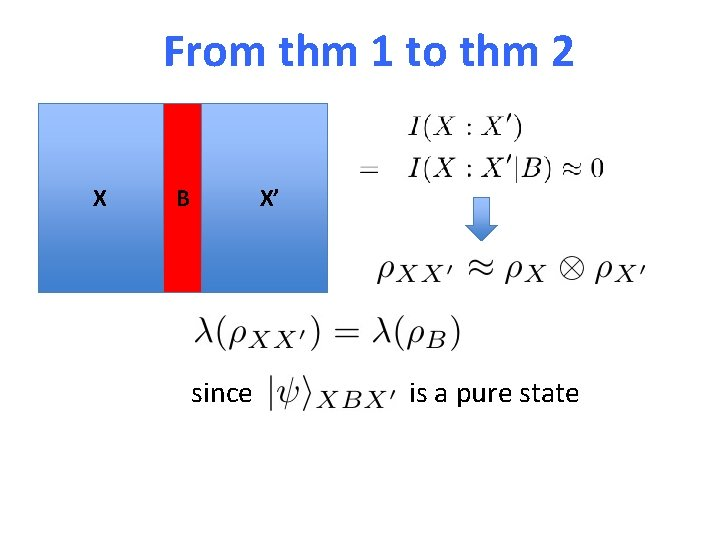From thm 1 to thm 2 X B X’ since is a pure state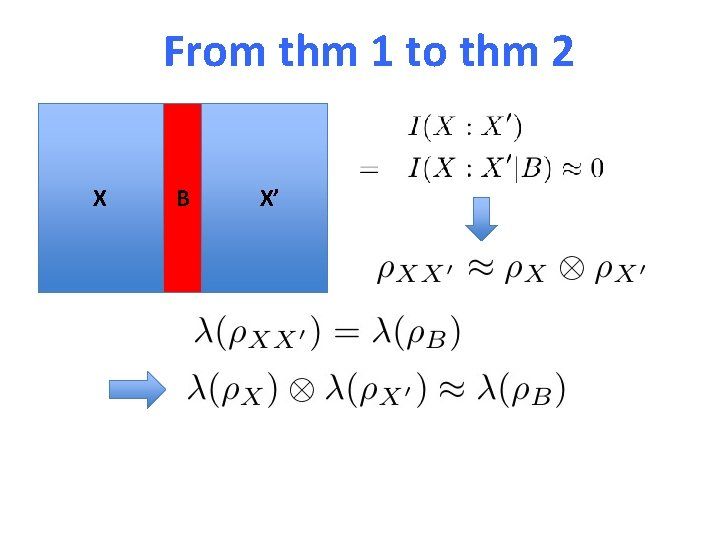From thm 1 to thm 2 X B X’From thm 1 to thm 2 X B If , X’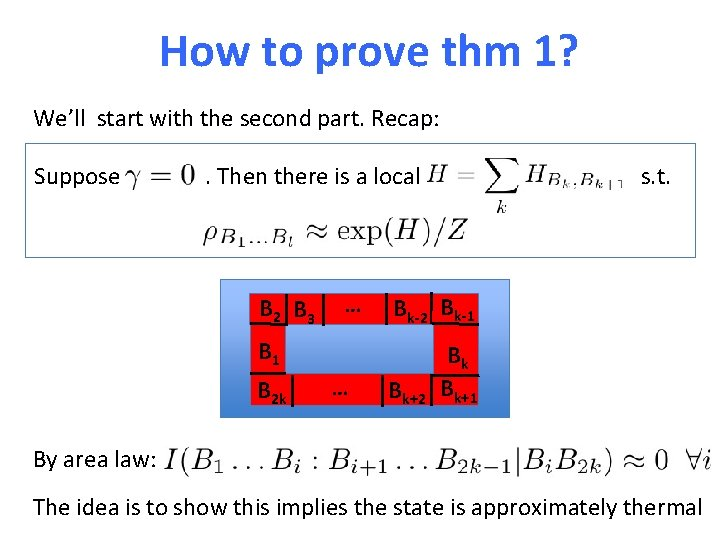How to prove thm 1? We’ll start with the second part. Recap: Suppose . Then there is a local s. t. B 2 B 3 … Bk-2 Bk-1 B 2 k … Bk+2 Bk Bk+1 By area law: The idea is to show this implies the state is approximately thermal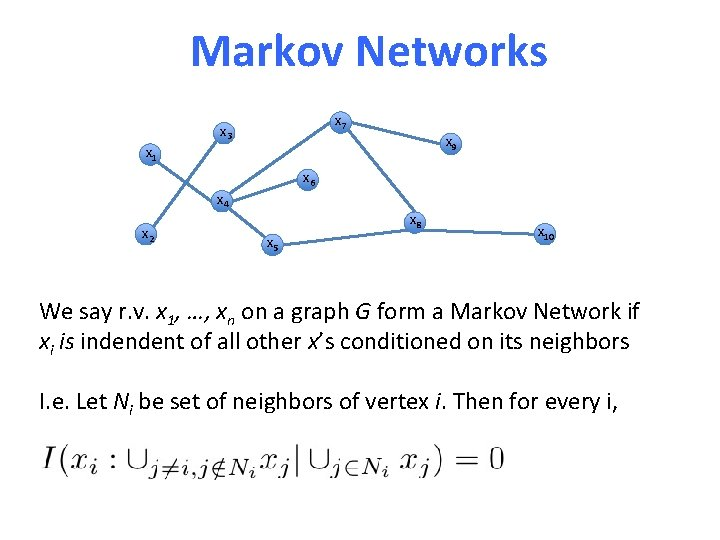Markov Networks x 7 x 3 x 9 x 1 x 6 x 4 x 2 x 8 x 5 x 10 We say r. v. x 1, …, xn on a graph G form a Markov Network if xi is indendent of all other x’s conditioned on its neighbors I. e. Let Ni be set of neighbors of vertex i. Then for every i,Hammersley-Clifford Theorem x 7 x 3 x 9 x 1 x 6 x 4 x 2 x 8 x 5 x 10 (Hammersley-Clifford ‘ 71) Let G = (V, E) be a graph and P(V) be a positive probability distribution over r. v. located at the vertices of G. The pair (P(V), G) is a Markov Network if, and only if, the probability P can be expressed as P(V) = e. H(V)/Z where is a sum of real functions h. Q(Q) of the r. v. in cliques Q.Quantum Hammersley-Clifford Theorem q 7 q 3 q 9 q 1 q 6 q 4 q 2 q 8 q 5 q 10 (Leifer, Poulin ‘ 08, Brown, Poulin ‘ 12) An analogous result holds replacing classical Hamiltonians by commuting quantum Hamiltonians (obs: quantum version more fragile; only works for graphs with no 3 cliques) Can we get a similar characterization for general quantum thermal states?Approximate Quantum Hammersley-Clifford Theorem? A l B C Def: We say a quantum state is a (l, eps)approximate Markov network if for every regions ABC s. t. B shields A from C and B has width l, Conjecture: Approximate Markov Networks are equivalent to Gibbs states of general quantum local Hamiltonians (at least on regular lattices)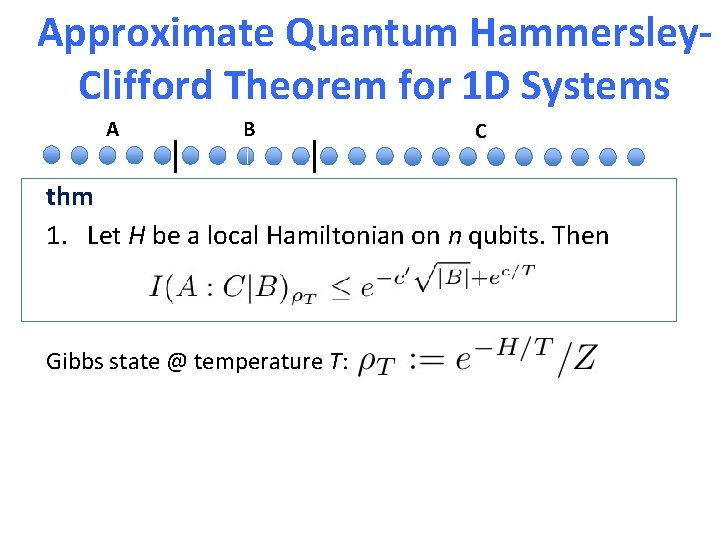Approximate Quantum Hammersley. Clifford Theorem for 1 D Systems A B C thm 1. Let H be a local Hamiltonian on n qubits. Then Gibbs state @ temperature T: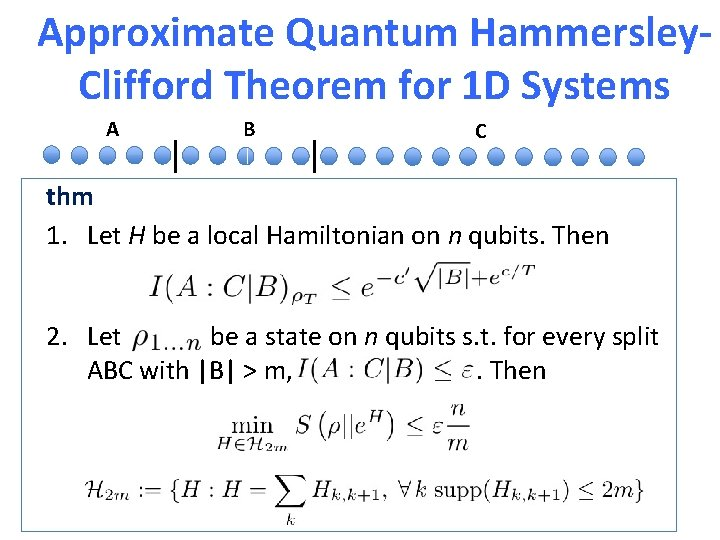Approximate Quantum Hammersley. Clifford Theorem for 1 D Systems A B C thm 1. Let H be a local Hamiltonian on n qubits. Then 2. Let be a state on n qubits s. t. for every split ABC with |B| > m, . Then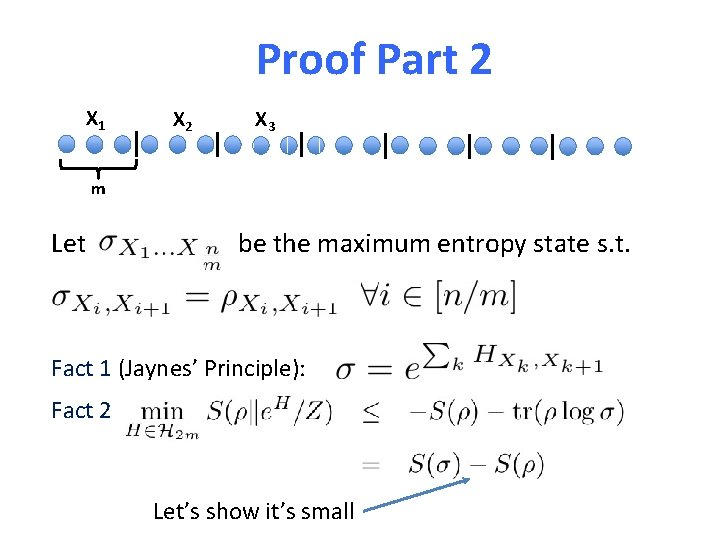Proof Part 2 X 1 X 2 X 3 m Let be the maximum entropy state s. t. Fact 1 (Jaynes’ Principle): Fact 2 Let’s show it’s smallProof Part 2 X 1 m SSA X 2 X 3Proof Part 2 X 1 m X 2 X 3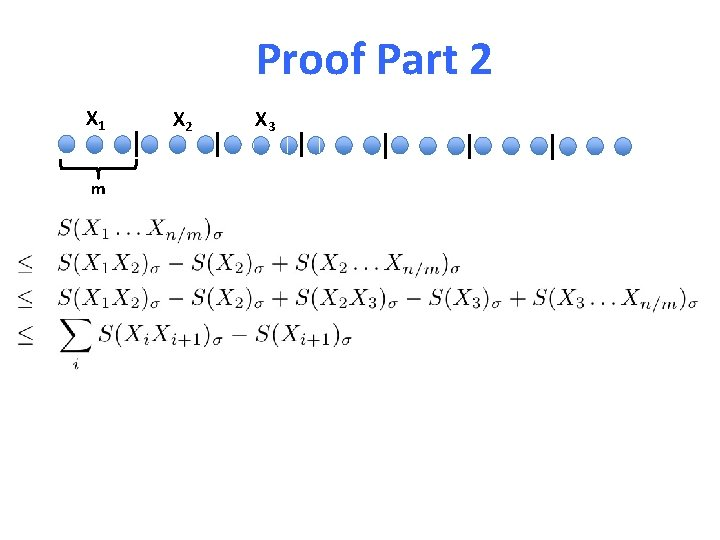Proof Part 2 X 1 m X 2 X 3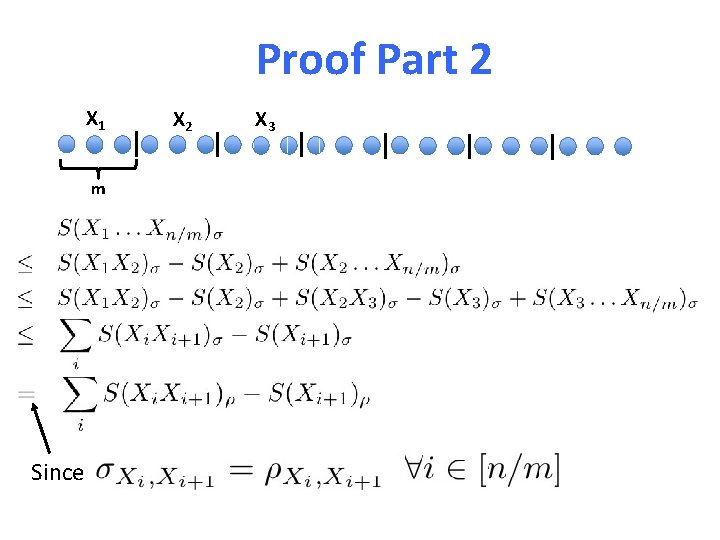Proof Part 2 X 1 m Since X 2 X 3Proof Part 2 X 1 m Since X 2 X 3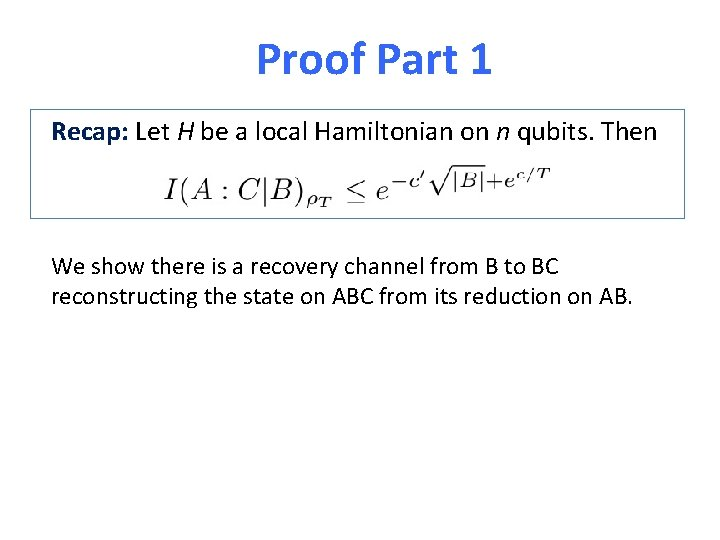Proof Part 1 Recap: Let H be a local Hamiltonian on n qubits. Then We show there is a recovery channel from B to BC reconstructing the state on ABC from its reduction on AB.Structure of Recovery Map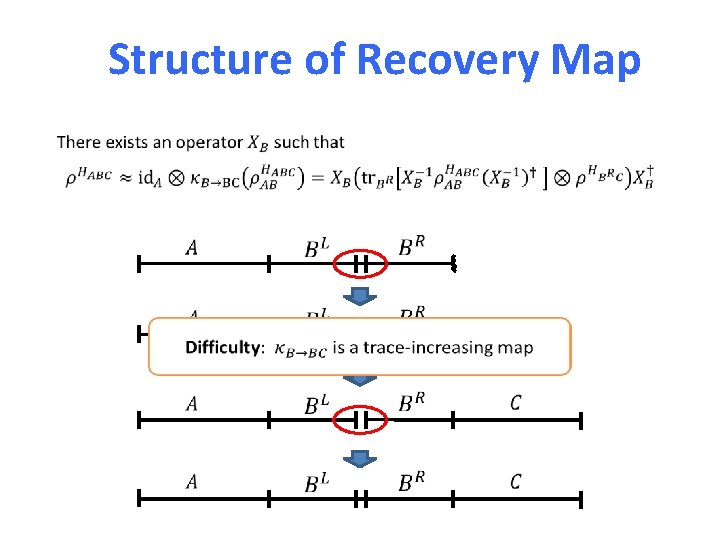Structure of Recovery Map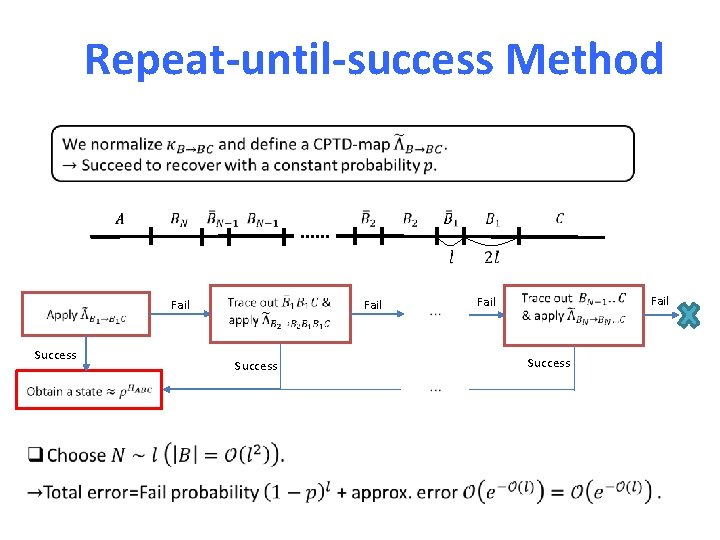Repeat-until-success Method Success Fail SuccessLocality of Perturbations Local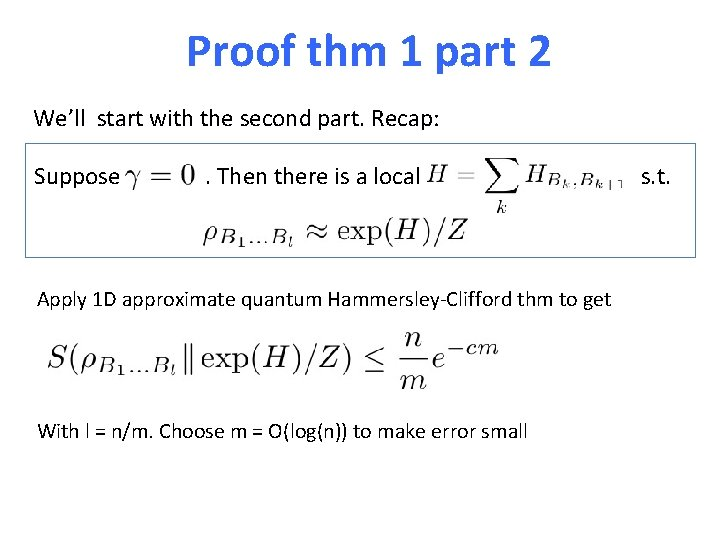Proof thm 1 part 2 We’ll start with the second part. Recap: Suppose . Then there is a local s. t. Apply 1 D approximate quantum Hammersley-Clifford thm to get With l = n/m. Choose m = O(log(n)) to make error smallProof thm 1 part 1 thm 1 Suppose satisfies the area law assumption. Then A B B C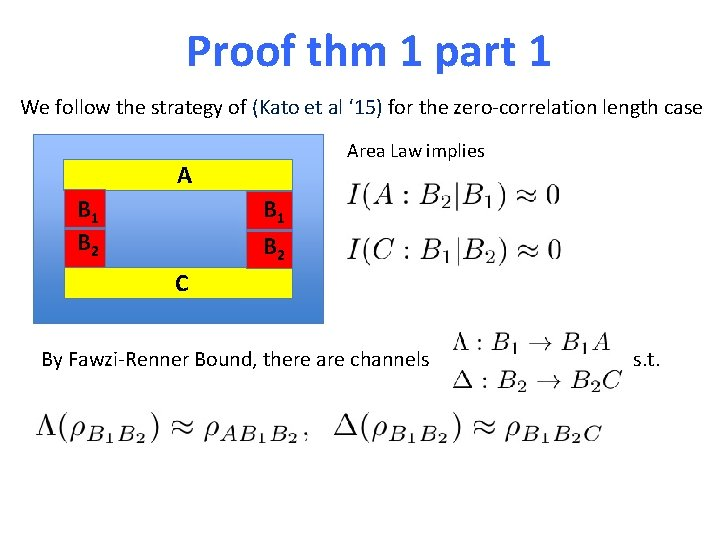Proof thm 1 part 1 We follow the strategy of (Kato et al ‘ 15) for the zero-correlation length case Area Law implies A B 1 B 2 C By Fawzi-Renner Bound, there are channels s. t.Proof thm 1 part 1 Define: We have It follows that C can be reconstructed from B. Therefore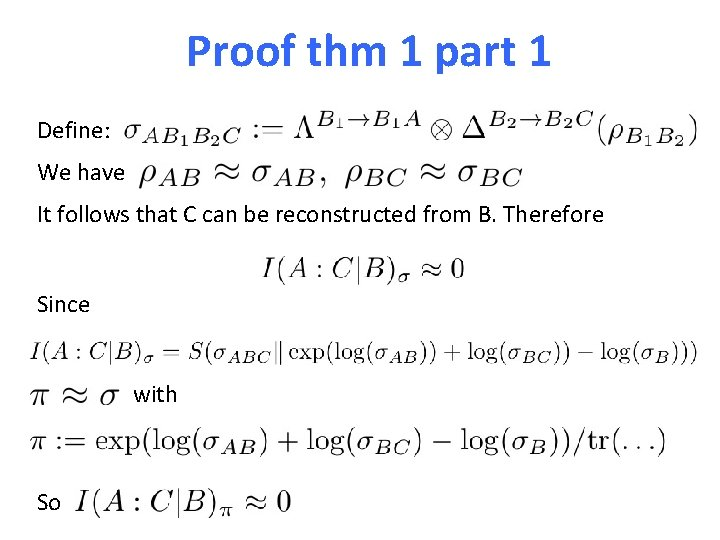Proof thm 1 part 1 Define: We have It follows that C can be reconstructed from B. Therefore Since with SoProof thm 1 part 1 Since Let R 2 be the set of Gibbs states of Hamiltonians H = HAB + HBC. ThenOpen Problems • What happens in dim bigger than 2? • Can we prove the approximate Markov property for general quantum states? • Can we prove the converse, i. e. that approximate quantum Markov Networks are approximately thermal? • Are two copies of the entanglement spectrum necessary to get a local boundary model? Thanks!